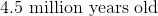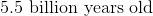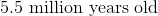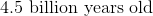Example Questions

← Previous 1

Example Question #1 : 8th Grade Science

Select the type of force that is being described:

A person being kept on the ground.

Applied force

Gravity force

Normal force

Friction force

Gravity force

Explanation:

There are many types of forces, but our answer choices include four types. In order to answer this question correctly, we need to be able to decipher between them.

Applied force is a force that is applied by a person to an object.

Gravity force is also known as the weight of the object. It's the force that allows a large object to attract another object towards itself.

Normal force is also known as supportive force. It is the force exerted upon an object that is in contact with another object, one object holds the other up.

Friction force is the force exerted by a surface as an object tries to moves across the surface.

Let's think about the scenario from the question. What causes a person to be kept on the ground, rather than floating around? Gravity forces us towards the ground; thus, gravity force is the correct answer.

Example Question #1 : 8th Grade Science

Select the type of force that is being described:

A plate sitting on a table top.

Normal force

Applied force

Gravity force

Friction force

Normal force

Explanation:

There are many types of forces, but our answer choices include four types. In order to answer this question correctly, we need to be able to decipher between them.

Applied force is a force that is applied by a person to an object.

Gravity force is also known as the weight of the object. It's the force that allows a large object to attract another object towards itself.

Normal force is also known as supportive force. It is the force exerted upon an object that is in contact with another object, one object holds the other up.

Friction force is the force exerted by a surface as an object tries to moves across the surface.

Let's think about the scenario from the question. A plate is sitting on the table, they are in contact with one another and the table is keeping the plate from falling to the ground; thus, this is an example of normal force.

Example Question #3 : 8th Grade Science

Select the type of force that is being described:

A marble rolling across the floor.

Normal force

Gravity force

Friction force

Applied force

Friction force

Explanation:

There are many types of forces, but our answer choices include four types. In order to answer this question correctly, we need to be able to decipher between them.

Applied force is a force that is applied by a person to an object.

Gravity force is also known as the weight of the object. It's the force that allows a large object to attract another object towards itself.

Normal force is also known as supportive force. It is the force exerted upon an object that is in contact with another object, one object holds the other up.

Friction force is the force exerted by a surface as an object tries to moves across the surface.

Let's think about the scenario from the question. We have a marble, an object, that is rolling across the floor, which is a surface. Based on the definitions of the types of forces above, friction force is the correct answer.

Example Question #4 : 8th Grade Science

Select the type of force that is being described:

A student pushing the teacher's desk across the room.

Friction force

Gravity force

Normal force

Applied force

Applied force

Explanation:

There are many types of forces, but our answer choices include four types. In order to answer this question correctly, we need to be able to decipher between them.

Applied force is a force that is applied by a person to an object.

Gravity force is also known as the weight of the object. It's the force that allows a large object to attract another object towards itself.

Normal force is also known as supportive force. It is the force exerted upon an object that is in contact with another object, one object holds the other up.

Friction force is the force exerted by a surface as an object tries to moves across the surface.

Let's think about the scenario from the question. We have a student pushing a desk, which is an object, across the room. The student would have to apply some force to get the desk to move. Looking at the types of forces above, applied force fits this scenario best.

Example Question #1 : Earth And Space Science

Roughly, how old is the planet Earth?Explanation:

Planet Earth is roughlyExample Question #2 : Earth And Space Science

What orbits the Sun?

Neither the Earth or the moon

The moon

The Earth

Both the Earth and the moon

The Earth

Explanation:

The Earth orbits the sun.

Example Question #3 : Earth And Space Science

What orbits the Earth?

The moon

Neither the moon or the sun

The sun

Both the moon and then sun

The moon

Explanation:

The moon orbits the Earth.

Example Question #2 : 8th Grade Science

Select the option that is an example of a renewable energy source.

Petroleum

Coal

Wind

Natural gas

Wind

Explanation:

Renewable energy sources are sources of energy that are naturally replaced or energy sources that won't run out.

Wind is the only example of an energy source that won't run out and is the correct answer.

Example Question #1 : Life Science

What is the process that enables organisms that are best suited for the environment to live and pass on their genetic traits.

Mutation

Environmental Selection

Evolution

Natural Selection

Natural Selection

Explanation:

Natural Selection is the process that enables organisms that are best suited for the environment to live and pass on their genetic traits.

Example Question #2 : Life Science

What is characterized as a mistake or change in an organism's DNA?

Evolution

Environmental Selection

Natural Selection

Mutation